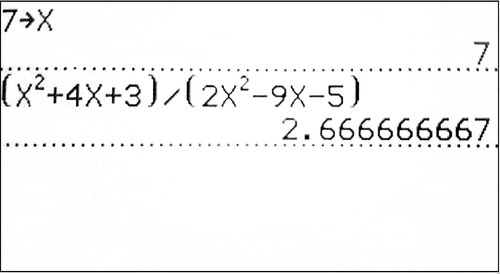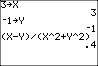# 1.5.6: Calculator Use with Algebra Expressions

•• Contributed by CK12
• CK12

## Calculator Use with Algebraic Expressions

What if you wanted to evaluate the expression (x2+4x+3)/(2x2−9x−5) when x=7? If you had your calculator handy, it could make things easier, but how would you enter an expression like this into your calculator? Also, how would you tell your calculator that x=7?

### Using a Calculator to Evaluate Expressions

A calculator, especially a graphing calculator, is a very useful tool in evaluating algebraic expressions. A graphing calculator follows the order of operations, PEMDAS. In this section we will explain two ways of evaluating expressions with a graphing calculator.

Method 1 This method is the direct input method. After substituting all values for the variables, you type in the expression, symbol for symbol, into your calculator.

Method 2 This method uses the STORE function of the Texas Instrument graphing calculators, such as the TI-83, TI-84, or TI-84 Plus.

#### Let's use a graphing calculator to solve the following problems:

1. Evaluate [3(x2−1)2−x4+12]+5x3−1 when x=−3.

First, try Method 1:

Substitute the value x=−3 into the expression.

[3((−3)2−1)2−(−3)4+12]+5(−3)3−1

Now, type it directly into the calculator as shown below:

The potential error here is that you may forget a sign or a set of parentheses, especially if the expression is long or complicated. Make sure you check your input before writing your answer. An alternative is to type in the expression in appropriate chunks – do one set of parentheses, then another, and so on.

To compare, let's also try Method 2:

First, store the value x=−3 in the calculator. Type -3 [STO] x. (The letter x can be entered using the x-[VAR] button or [ALPHA] + [STO]). Then type in the expression in the calculator and press [ENTER].

Note: On graphing calculators there is a difference between the minus sign and the negative sign. When we stored the value negative three, we needed to use the negative sign, which is to the left of the [ENTER] button on the calculator. On the other hand, to perform the subtraction operation in the expression we used the minus sign. The minus sign is right above the plus sign on the right.

1. Evaluate the expression: 3x2−4y2+x4(x+y)12 for x=−2,y=1.

Store the values of x and y: −2 [STO] x, 1 [STO] y. The letters x and y can be entered using [ALPHA] + [KEY]. Input the expression in the calculator. When an expression shows the division of two expressions, be sure to use parentheses: (numerator) ÷ (denominator). Press [ENTER] to obtain the answer −.88 or −89.

### Examples

Example $$\PageIndex{1}$$

Earlier, you were asked to evaluate the expression (x2+4x+3)/(2x2−9x−5) when x=7. To tell the calculator that x=7, store the value of x.

SolutionType in 7 [STO] x to store the value of x. The letter x can be entered using [ALPHA]+[KEY]. Then input the expression in the calculator. Make sure to use parentheses to group the expressions in the numerator and denominator together. Press [ENTER] to obtain the answer 2.6 or 8/3.

Example $$\PageIndex{1}$$

Evaluate the expression (x−y)/(x2+y2) for x=3,y=−1.

SolutionStore the values of x and y: 3 [STO] x, -1 [STO] y. The letters x and y can be entered using [ALPHA] + [KEY]. Input the expression in the calculator. When an expression shows the division of two expressions, be sure to use parentheses: (numerator) ÷ (denominator). Press [ENTER] to obtain the answer 0.4.

### Review

In 1-5, evaluate each expression using a graphing calculator.

1. x2+2x−xy when x=250 and y=−120
2. (xy−y4)2 when x=0.02 and y=−0.025
3. (x+y−z)/(xy+yz+xz) when x=1/2, y=3/2, and z=−1
4. (x+y)2/(4x2−y2) when x=3 and y=−5d
5. The formula to find the volume of a spherical object (like a ball) is V=(4(π)r3)/3, where r= the radius of the sphere. Determine the volume for a grapefruit with a radius of 9 cm.

In 6-9, insert parentheses in each expression to make a true equation.

1. 5−2⋅6−4+2=5
2. 12÷4+10−3⋅3+7=11
3. 12−8−4⋅5=−8
4. Let x=−1. Find the value of −9x+2.